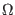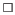Next: Switches Up: Passive Element Lines Previous: Resistors   Contents   Index

## Resistor Model

Type Name: r

The resistor model consists of process-related device data that allow the resistance to be calculated from geometric information and to be corrected for temperature. The parameters (multiple names are aliases) available are:

 name Resistor Model Parameters parameter units default example m parallel multiplier - 1.0 1.2 tc1, tc, tc1r first order temperature coeff./C 0.0 - tc2, tc2r second order temperature coeff./C2 0.0 - rsh sheet resistance/- 50 defl, l default length M 0 2e-6 defw, w default width M 0 2e-6 dl, dlr length reduction due to etching M 0 1e-7 narrow, dw narrowing due to side etching M 0 1e-7 tnom, tref parameter measurement temperature C 25 50 temp default instance temperature C 25 50 kf flicker noise coefficient 0 af flicker noise exponent of current 2 ef flicker noise exponent of frequency 1 wf flicker noise exponent of width 1 lf flicker noise exponent of length 1 noise noise conductance multiplier 1

The sheet resistance is used with the etch reduction parameters and l and w from the resistor element line to determine the nominal resistance by the formula

R = rsh . (l - dl)/(w - narrow).

The parameters defw and defl are used to supply default values for element w and l if not specified on the device line. A fatal error is produced if the resistance can't be determined from given parameters.

After the nominal resistance is calculated, it is adjusted for temperature by the formula:

R(temp) = R(tnom) . (1 + tc1 . (temp- tnom) + tc2 . (temp- tnom)2 )

Finally, the resistance is divided by the parallel multiplier (m) value.

The flicker noise capability can be used in noise analysis. This requires that kf, l, and w be specified. To use, the instance line must reference a model, but also can have a resistance specified which will override model calculation of resistance.

Flicker noise model:

Noise = (KF . IAF)/(LeffLF . WeffWF . fEF)

Param Description Units
Noise Noise spectrum density A2 Hz
I Current A
Leff Eff length (L-DL) M
Weff Eff width (W-DW) M
f Frequency Hz
Param Description Default, Range
KF Flicker noise coefficient 0, > = 0
AF Exponent of current 2, > 0
LF Exp. of eff. length 1, > 0
WF Exp. of eff. width 1, > 0
EF Exp. of frequency 1, > 0

The noise parameter will multiply the conductance used in the noise equations. It provides a default which is overridden by the instance parameter of the same name. This can be used to model empirical excess noise, or to remove the devices from noise analysis by setting the parameter to zero.Next: Switches Up: Passive Element Lines Previous: Resistors   Contents   Index
Stephen R. Whiteley 2022-09-18